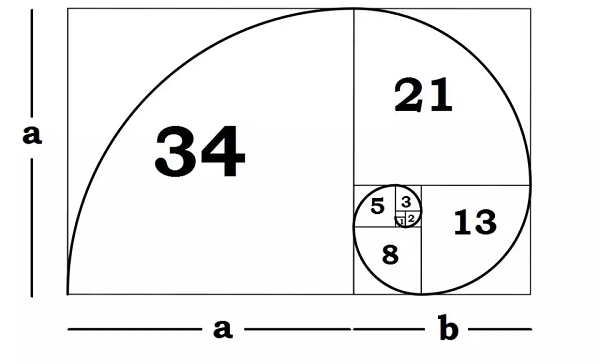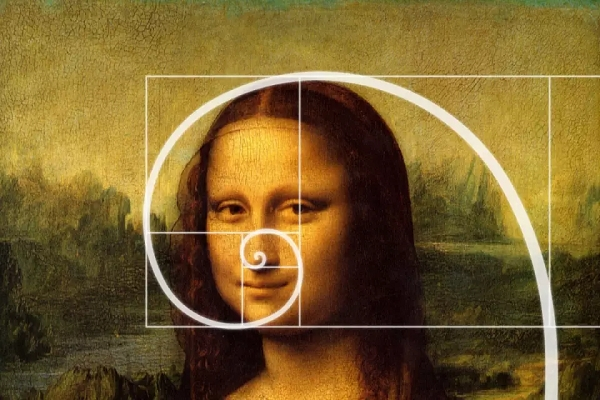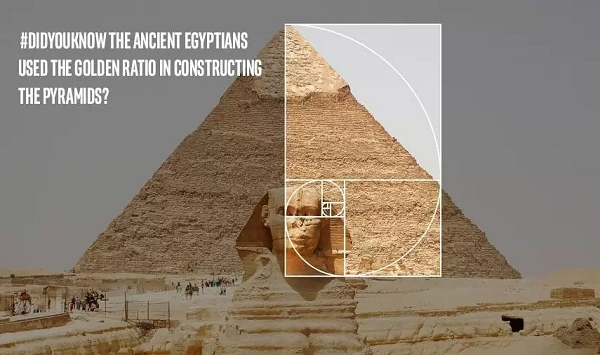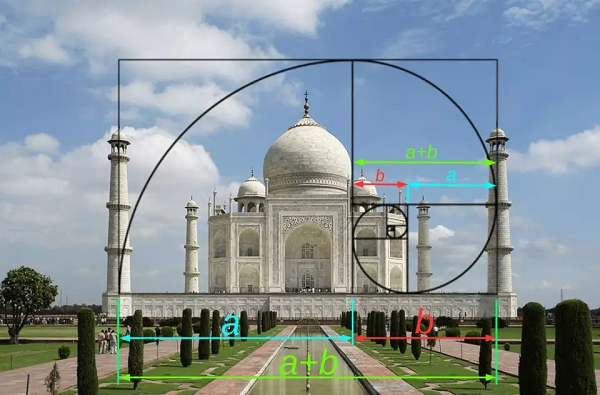# What is the link between Phi and the Golden Ratio?

Phi is the basis for the Golden Ratio, Section or Mean. The value of Phi ( Φ ) is 1.618033988749895. It has many unusual mathematical properties.

The Golden ratio is a special number found by dividing a line into two parts so that the longer part divided by the smaller part is also equal to the whole length divided by the longer part. It is often symbolized using phi, after the 21st letter of the Greek alphabet.The ratio or proportion, determined by Phi (1.618 …) was known to the Greeks as the “dividing a line in the extreme and mean ratio” and to Renaissance artists as the “Divine Proportion” It is also called the Golden Section, Golden Ratio, and the Golden Mean.

There are some classic examples of Golden Ratio.

## The Monalisa Painting

Monalisa's Face is a perfect Golden Rectangle according to the ratio of the width of her forehead compared to the length of the top of her forehead to the chin.## The Great Egyptian Pyramids## The Taj Mahal## Nature